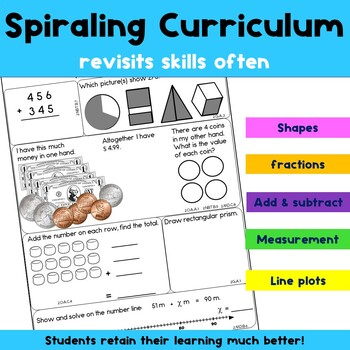# Daily Math for Second Grade Month 6Subject
Resource Type
File Type

PDF

(3 MB|28 pages)
Standards
Also included in:
1. Perfect for morning work or math centers, this growing year long bundle of daily math resources will have your second graders engaged in reviewing many math skills! When completed it will includes 180 pages of spiraling math practice, designed for 180 days of second grade, with all instructions and
\$40.00
\$35.00
Save \$5.00
• Product Description
• StandardsNEW

Perfect for morning work or math centers, this daily math resource will have your second graders engaged in reviewing many math skills! It includes 20 pages, designed for the sixth month of second grade, with all instructions and answer keys included, and each problem is labeled with CCSS for math! You'll love how easy it is to cover the CCSS for Math with this NO PREP resource!

With this resource your students will:

• Solve two step word problems with answers to 100 with regrouping
• Count by 1's, 2's, 5's and 10's to find missing numbers within 1000
• Use repeated addition to find total units in an array (up to 5x5)
• Compare 3 digit numbers with < = >
• Identify and represent numbers as groups of 100s, 10s and 1's
• Work with standard and expanded form of 3 digit numbers
• Add and subtract four 2-digit numbers within 100 with regrouping
• Add and subtract 2 3-digit numbers within 1000 with regrouping
• Mentally +10, -10, +100, -100 within 1000
• Explain why addition and subtraction strategies work
• Measure inches, centimeters and millimeters and choose appropriate tools to measure
• Measure the same object with different units, describe how the answers relate to the size of the unit of measure
• Estimate length in millimeters, centimeters, inches and feet
• Solve word problems with a symbols for the unknown in the problem
• Find sums and differences within 100 on a number line
• Tell time to 5 minute intervals
• Count money with pennies, nickels, dimes, quarters, \$1 & \$5 bills
• Use repeated measurement to create and read line plots
• Create and read bar graphs (up to 4 categories) and interpret data
• Identify and draw shapes
• Divide rectangles and circles into halves, thirds and quarters, understand that equal shares of identical wholes may not have the same shape.
• Partition rectangles into rows and columns and determine how many smaller units that creates (up to 5 rows x 5 columns)

• "My students enjoyed this daily math resource. The activities and skills varied from day to day, so their interest level was high. The format was very readable for them, too. It was also a nice tool for me to see where they needed extra practice."

You may also like:

Thank you for visiting Paula's Primary Classroom. All new resources are 50% off for the first 48 hours. To be notified when new activities become available, and of upcoming sales, please click on the green star below my store name and follow me. Followers and feedback are greatly appreciated!

Click here to be notified when new resources are released at 50% off!

Have a great day!

♥ Paula

Partition circles and rectangles into two, three, or four equal shares, describe the shares using the words halves, thirds, half of, a third of, etc., and describe the whole as two halves, three thirds, four fourths. Recognize that equal shares of identical wholes need not have the same shape.
Partition a rectangle into rows and columns of same-size squares and count to find the total number of them.
Recognize and draw shapes having specified attributes, such as a given number of angles or a given number of equal faces. Identify triangles, quadrilaterals, pentagons, hexagons, and cubes.
Use addition to find the total number of objects arranged in rectangular arrays with up to 5 rows and up to 5 columns; write an equation to express the total as a sum of equal addends.
Determine whether a group of objects (up to 20) has an odd or even number of members, e.g., by pairing objects or counting them by 2s; write an equation to express an even number as a sum of two equal addends.
Total Pages
28 pages
Included
Teaching Duration
1 month
Report this Resource to TpT
Reported resources will be reviewed by our team. Report this resource to let us know if this resource violates TpT’s content guidelines.